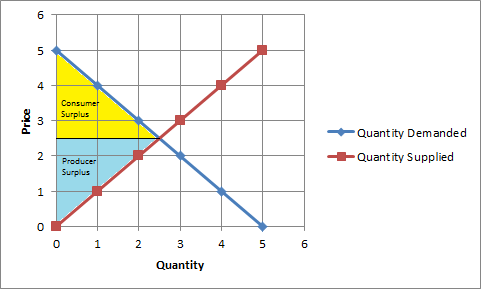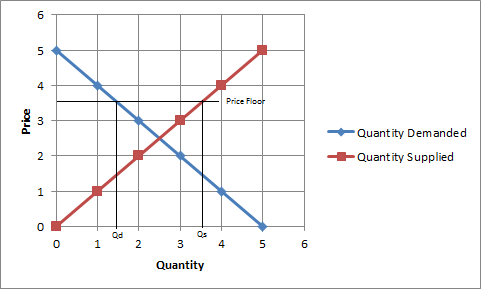In: Economics

# For Question 1-8, consider a competitive market for a good where the demand curve is determined...

For Question 1-8, consider a competitive market for a good where the demand curve is determined by the demand function: P=5-Q^ D and the supply curve is determined by the supply function: P=Q^S . Where P stands for Price, Q^D is quantity demanded and Q ^S is quantity supplied.

1. What is the quantity demanded of the good when the price level is P = $1? 2. What is the quantity supplied of the good when the price level is P =$1?

3. At P=$1 there is: a. Competitive equilibrium in the market; b. Excess supply in the market; c. Excess demand in the market; d. Rationing in the market; c. Excess demand in the market; 4. What is the equilibrium quantity level for the good in the competitive market? 5. What is the equilibrium price level for the good in the competitive market? 6. What is the consumer surplus in the competitive market? 7. What is the producer surplus in the competitive market? 8. What is the total surplus in the competitive market? For Question 9-13, assume a market intervention of the form of price floor. The price floor is set at P=$3.5. This price floor is binding, so it has an impact on the equilibrium of the economy.

9. How many units of the good the producers are willing to supply the market at the considered market intervention?

10. How many units of the good the consumers are willing to demand the market at the considered market intervention?

11. At price $3.5 there is: a. Competitive equilibrium in the market; b. Excess supply in the market; c. Excess demand in the market; d. Rationing in the market; 12. What is the value of the Consumer Surplus considering this market intervention? 13. What is the value of the Producer Surplus considering this market intervention? ## Solutions ##### Expert Solution Given, P = 5-Qd => Qd = 5-P P = Qs 1. When P =$1, Qd = 5-P = 5-1 = 4

2. When P = $1, Qs = P = 1 3. At P =$1, Qs = 1 and Qd = 4. As the quantity demanded is greater than the quantity supplied, there is a shortage (excess demand) in the market.

Ans: c. Excess demand in the market

4. At equilibrium, Qd = Qs

=> 5-P = P

=> 5 = P+P

=> P = 2.5

Qs = Qd = 2.5

Equilibrium quantity = 2.5 units

5. Equilibrium price level = P = $2.5(as obtained in Q4) 6.Consumer Surplus = Area of the shaded triangle in the above graph = 1/2 x base x height = 0.5 x 2.5 x 2.5 =$3.125

7. Producer Surplus = 1/2 x base x height = 0.5 x 2.5 x 2.5 = $3.125 8. Total Surplus = Consumer Surplus + Producer Surplus =$3.125 + $3.125 =$6.25

9.9, 10 & 11: